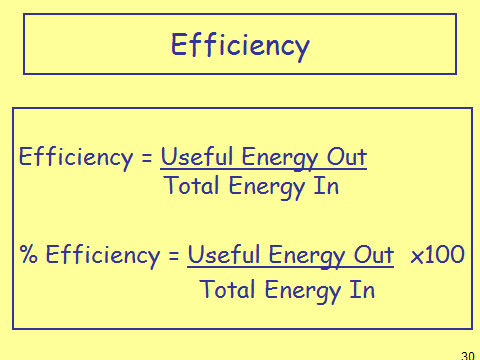# Efficiency

## What Is Efficiency

Efficiency is a measure of how well an object outputs useful energy, compared to how much energy is taken in. The measure is between 0 and 1, where 1 is totally efficient. This can also be measured between 0% and 100% and is done by multiplying your answer by 100.

## Efficiency CalculationsSometimes you will be asked to leave your answer as a decimal (first equation) and sometimes as a percentage (second question). It is important to read the question carefully to see which one it wants. If it is unspecified then you can leave your answer in either form.

## Improving Efficiency

Sometimes you will be asked how to improve efficiency. Like we saw last lesson, we must increase the amount of useful energy and by so doing decrease the amount of wasted energy. We can do this a number of ways such as reducing friction or by increasing the amount of insulation around an object or material.

What is the efficiency of bulb A which has 500J input and 75J of useful output?